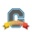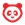# Community

cancel
Showing results for
Search instead for
Did you mean:

# Understanding how grades are calculated when using Assignment GroupsCommunity Coach
12 11 21.7K

The easiest way to set up percent based (or weighted) grading for your Canvas course is to use groups on the Assignments page. Assignment groups are used to show how the different assignments in your course are weighted differently. Yet, there are some important considerations and things you should understand when you go to set up your Assignment Groups and Assignments.

• Individual assignments within an Assignment Group do NOT count equally, it depends on the number of points an assignment is worth. In other programs (like WebAssign) there is an option you can check to weight all assignments equally. Canvas does not have this option. Within an Assignment Group an assignment worth 100 points will be weighted and worth more than a 25 point assignment.
• If you want assignment grades to be weighted equally within an Assignment group here are the options:
• Separate each assignment (quiz, etc) into their own Assignment Group and assign each group the same weight. This might work well if you don't have too many assignments, but if you have a lot of assignments this could definitely get crazy!
• Make each assignment in the Assignment Group worth the same number of points. Example: All assignments in the HW group are worth 20 points.
• Instructor adjusts grade before entering it into Canvas. If students aren't submitting assignments in Canvas (they are turning it in on paper) then the instructor can adjust the grades before entering them. Example: the instructor grades the HW and the student gets 17/20 points or 85%. In Canvas the instructor enters 85 for the HW grade (out of 100) instead of the 17/20. Next homework the student gets 15/25 or 60% so the instructor enters 60 for the HW grade instead of 15 (for the 15/25). Note this definitely isn't the best work around and wouldn't work at all for quizzes or other assignments submitted in Canvas.
• Why is the grade calculated this way? Mathematically this is the way weighted averages work. See example below for how this works.
• (total points a student has earned from assignments in a group)/(total points possible in the group) = percent that shows up for that group in the Canvas gradebook
• If a student earned 10/25 points for HW1 and 90/100 points for HW2 this is how their grade would be calculated within the Homework Assignment Group --> (Student earned HW 1 points + Student earned HW2 points)/(HW1 + HW2 total points possible) = (10+90)/(25+100) = .80 or 80% = student's grade for the Homework Assignment Group.

Here are a few useful guides that might help as well:

I know this can definitely be confusing, but I hope it helps!

Tags (3)
11 CommentsCommunity Team

Great resource,  @kona ! It's worth noting that Canvas Quizzes can only be calculated on the basis of points; currently, they cannot be expressed as percentages. So, if your grading scheme calls for all quizzes of a certain type to contribute equally to the final course grade, you must either make them all worth the same number of points or put each of them in its own assignment group, each of which carries the same weighting (I opt to make each of my quizzes worth 100 points so that they mimic percentages in the eyes of the students, but this isn't always feasible).Community Participant

Is there an easy way to make quizzes worth 100 points when the number of questions in each varies? Or do you have to adjust the point value of each question so that everything adds up to 100?Community Team

@ghalsell , at the moment, it's the latter. You will have to tinker with the point values of each question so that everything adds up to 100. There's a relatively easy way to do this, though, if you'd like to have the order of your questions randomized, and you are willing to assign the same point value to various questions. If so, you can create a Question Group and drag all of the questions into that group. If you have 20 questions, you can then set the question group to assign five points per question to bring the quiz total up to 100.

Some resources for your consideration: How do I create a Quiz with a Question Group to randomize quiz questions?​ andCommunity Member

I recently found out that the grade computation methodology for Canvas is exactly what Kona Jones has written about in her post.

However, I respectfully beg to disagree on her statement

"Why is the grade calculated this way? Mathematically this is the way weighted averages work. See example below for how this works."

• Assume that I have  3 assignments to be completed by each student as part of their overall evaluation.
• Assume that these three assignments contribute to 15% of the final grade
• Assign 1 has three questions, Ass 2 has five and Ass 3 has seven questions
• For ease of grading I evaluate as follows:
• Ass 1: 2 points per question  x 3 questions = 6 points
• Ass 2: : 2 points per question x 5 = 10 points
• Ass 3: 2 points per question x 7 = 14

If the student gets 2 points on Ass1, 4 on Ass2  and 6 on Ass3, Canvas would grade this as (2 + 4 + 6)/30 = 40%

However, since each assignment has equal weightage in its own group, the correct mathematical computation would be:
(2/6 + 4/10 + 6/14)  * (1/3) = 38.73% which should be the corrected value

This may not seem like much of a difference but as the number of assignments in each group increase and the total points on which they are graded vary, the difference can be significant.

The total number of points for an assignment should not be an indicator of its weightage in the the total computation. Sometimes the points we use are for convenience. Some of our assignments have7 questions, some 10 questions, some 16 so we give points on a per-question basis.

The net result is that Canvas over-estimates the correct grade.

I guess, from a student point of view that is better than under-estimatingLooks like the only way to get the correct output is by making sure the total points is the same for all assignments within a group. And that is going to be a REAL PAIN.Community Contributor

Both of these mathematical computations could be "correct" and both could be "incorrect." It's two different ways of computing a grade. And, like most things, we have to use it the way it is programmed.

In addition, the existing way is the simplest way--assuming that the points are what you really want to score the students on. Assuming that you want everything in a group to be equally weighted is more complex (because you have to adjust it based on how many assignments there are in the group, rather than just adding everything up).

I work with faculty who for the most part do not want everything in a group to be equally weighted and put the points in based on how much relative worth each item is (say, the project is 100 points, and the drafts are 10 points each and selecting a topic is 1 point). So to me, the current way is "correct"!Community Member

I guess opinions differ on this. You work with faculty; I have been a faculty member for 40 years and in top universities. I teach Computer Science so my mathematics background is not too bad. No other faculty member I have worked with has ever used an approach where they compute the average as sum(numerators)/sum(denominators). That is wrong math and wrong methodology. Trying to justify the errors in Canvas computations is not going to fix the problem.

And believe me it is not a "complex problem"  as you point out. Canvas already computes percentages so the process of computing the correct average using the correct methodology is simply by computing the average of the percentage grades on individual assignments within an assignment group.

Maybe some instructors compute the averages the way Canvas is currently doing but I sure won't tell my students to compute the averages this way with denominators that are different.Community Participant

Hi Ramesh,

This is how I have grades calculated.

 Category Percentage (weight) Total Points # of submissions Papers 30% 300 2 Post Discussion 15% 150 10 Pre Discussion 15% 150 10 Final Exam 20% 200 1 Participation 2% 20 3 Analysis 18% 180 9 Total Points 1000

If a student got 300/300, are you saying the actual percentage is more than 30%?Community Contributor

I just reread your post and I think the difference in our views is: I don't think of category grades as "an average of the items in the category" but you do--hence your reference to computing averages. Rather, I think if it as a group of items that could total X points assignments. Each students gets some number of points on each assignment, total those points, and you get their points for the category.

Other LMSes (I just saw one the other day) allow you to select how you want a category to work. Perhaps a feature request for you to write up?

(P.S. I am also a faculty member and have a degree in math. I have seen faculty want it your way and those it want it the way Canvas does it. I work with whatever the LMS allows.)Community Member

That is definitely not the way how weighted average works mathematically, unless HW1 is exactly and precisely one fourth of the weight of HW2, which is nowhere assumed or stated (and almost never the case in real life teaching). I am very surprised how Canvas would not have an option for giving weights to individual assignments, or at least have the option to weigh them equally.Community Member

No he is not saying that as he is not talking about category weighting but individual assignment weighting. He is for instance talking about a case where your Papers category would have 2 papers but with difference impact levels at 60% and 40% but assigned 150 points each. In this case a score of 90 from the first and 60 from the second would register a 50% on Canvas. But the correct score with the impact you would like to have should be (90/150)*(60%)+(60/150)*(40%) = 36% + 16% = 52%. In other words instead of earning 156 points out of 300 points, Canvas is giving this student 150 points, and of course that impact the total percentage of the student.Community Team

Actually,  @dtunc ​, something along those lines may be in the works, and for additional information, I'll refer you to the conversations around these two feature ideas:

(it's archived, but received a high enough vote total from the Community to be placed on the product team's "dream list")

(currently open for voting, but with a rather anemic Community response thus far)